# Add Numbers in Excel Using Formula and Functions

In this tutorial, learn how to add numbers in Excel with formula or using functions. You can add two or more numbers in the Excel sheet using the method given here.

Use plus(+) operator or you can use `SUM()` the function to add numbers. Among all the method given here, the autosum is the fastest method to calculate addition in just a single click.

## Add Numbers in Excel Using Plus(+) Operator in Excel

1. Get How Many Numbers to Find
Identify the numbers first which you have to get the total. You can select each cell with a cell name that contains the numbers.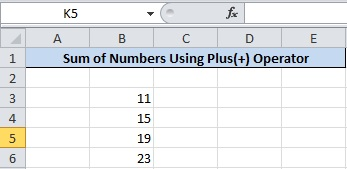There are four numbers given in the above image to calculate. The cell is from B3 to B6 which you have to use for calculation.

2. Use Equals to(=) operator to Calculate Total
Find the cell where you have to get the total of the numbers. In that cell, put the equals to(=) operator as the start of the formula.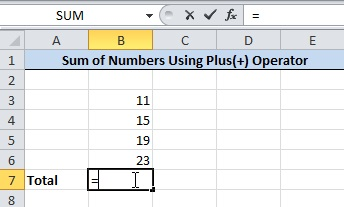The equals to(=) operator is showing the image given above.

3. Add Cell Name of All Number With Plus(+) Operator
You have to now write the names of all the cells contains the numbers. Also, add the plus(+) operator between the cell names.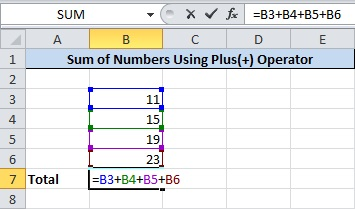The above image showing the added cell names after equals to (=) operator. The plus(+) operator is added between the names of each cell that contains the numbers.

4. Press Enter to Get Final Total of Numbers
After you have done writing the names of each cell with the plus(+) operator. Now, press the enter button of your keyboard to get the total. You will get the addition of the numbers in the cell as the final required result.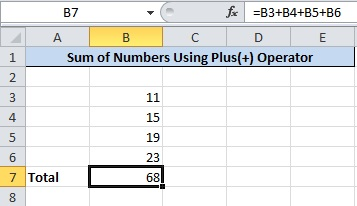The final total of all the numbers is as given in the image showing above. You can change or add more numbers to calculate the total as per requirement.

## Perform Addition of Numbers Using SUM Function in Excel

A function requires some variables to pass as an argument. The output of the function gives the result of some useful calculation. The calculation may be easy or difficult to find. However, Excel is a very powerful software which can calculate the most difficult calculation in minutes.

In order to find the addition of numbers in Excel with function. Excel comes with the `SUM()` function that calculates the addition of numbers. Follow the step-by-step process given below to use this function for calculation.

1. Use `=SUM` Where You Want to Find Total
Select the cell where you want to find the total of the number. In that cell, put `=SUM` to start using the function.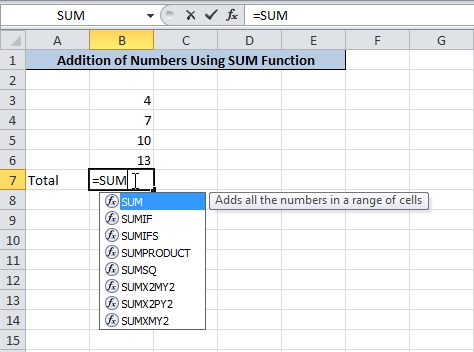Use the above-given image to understand how to put the function in the cell.

2. Put Start Bracket After SUM
Start bracket is required to put after the above step. After you enter the bracket, the variables in the format of numbers start showing as given in the image below. These numbers are the arguments of the function to add in comma separation.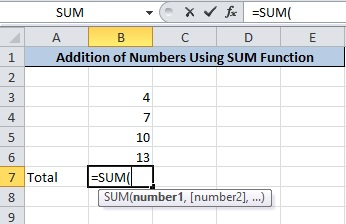The above image showing the arguments as `number1, [number2],...` to pass. Check the next step to enter your numbers as the argument of the function.

3. Select Range of All Numbers Cells
Now, select all the cells that contain the numbers. It can be in sequence of range as showing in the image below. You can also select the random cells to add as arguments.

When you complete selecting the cells, the cell names are starts showing within the brackets.The above example showing the selected range of cells from B3 to B6. The cell range showing as `B3:B6` inside the function brackets.

4. Put Close Bracket After Cell Names
Place a closing bracket at the end of the cell range name. The closing bracket completes the function with the arguments.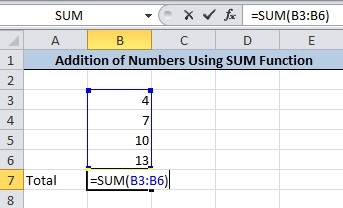After you enter the closing brackets, your function will appear as the image given above.

5. Press Enter to Get Final Output
If you have completed following all the above steps. Just press the enter button to get the calculated given as the output.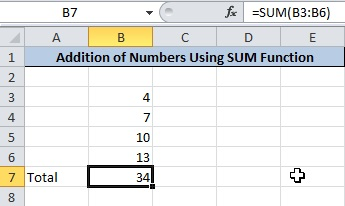An output showing in the above image is the total of the numbers in range. If you have no idea about cell range, you can check our post based on cell range in Excel.

## Autosum Selected Cell to Get Sum of Numbers in Excel

In addition to above all, an autosum method is also available to get total in just single click. Calculate total in Excel sheet with this fastest method in seconds.

1. Enter Numbers in Cells To Calculate Total
First of all, place number which you want to calculate the total. If you already have the number in the Excel Sheet. Just follow the next step given below.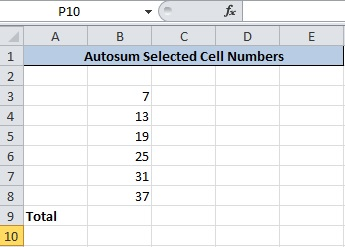Let’s take an example of the numbers given in the above image.

2. Select Cells Contains Numbers To Find
You don’t have to go to the cell where you want to get the total. Just select all the numbers to calculate total in the result.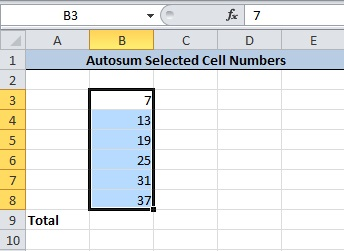The above image showing all the selected numbers whose total is to calculate.

3. Click ‘AutoSum’ Given Under Home>>AutoSum
This tutorial is based on MS Excel 2010. Click the ‘Home’ menu option given at the top of the Excel Sheet. Under this menu, there are various options from which you have to click the ‘Σ AutoSum’ option.

This is the option which calculates the total of the selected numbers in Excel Sheet.The above image showing the ‘Σ AutoSum’ option given under Home >> AutoSum.

4. Final Sum Output
After you click the ‘Σ AutoSum’ option in the above step. You will get the final calculated total as the image given below.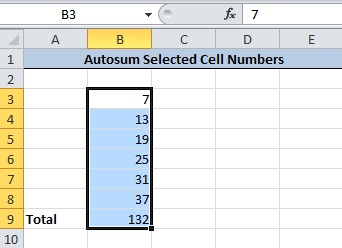The total of the numbers comes just below the selected numbers.

If you want to learn the above given methods with video tutorial. Just Click the video given below.

Hope, you like this post of how to add numbers in Excel using formula and functions. If you have any query regarding the tutorial, please comment below.

Also tell me, which method you are using to find the total of the number in the Excel Sheet.## THE ULTIMATE TOOLKIT

Get FREE Access to Toolkit and Resources that we are using in Tutorialdeep that Every Professional Should Have! in one handy PDF.